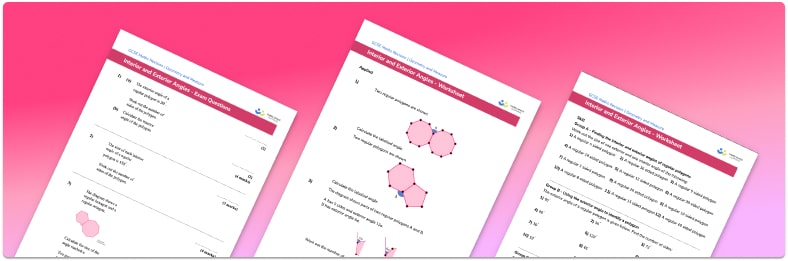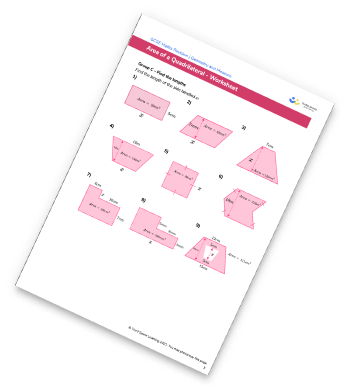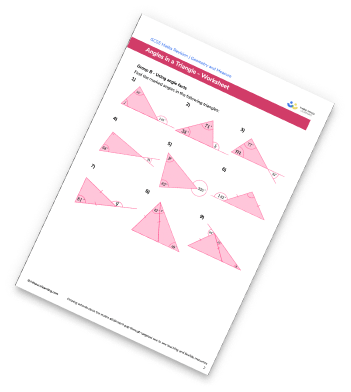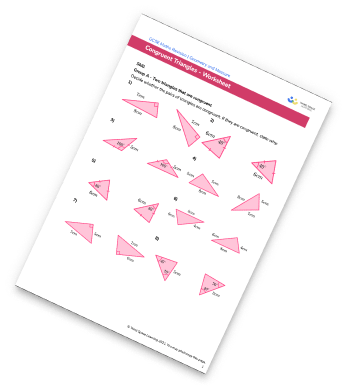# Interior and Exterior Angles Worksheet• Section 1 of the interior and exterior angles worksheet contains 20+ skills-based interior and exterior angles questions, in 3 groups to support differentiation
• Section 2 contains 3 applied interior and exterior angles questions with a mix of worded problems and deeper problem solving questions
• Section 3 contains 3 foundation and higher level GCSE exam style questions on interior and exterior angles
• Answers and a mark scheme for all interior and exterior angles questions are provided
• Questions follow variation theory with plenty of opportunities for students to work independently at their own level
• All questions created by fully qualified expert secondary maths teachers
• Suitable for GCSE maths revision for AQA, OCR and Edexcel exam boards

• This field is for validation purposes and should be left unchanged.

You can unsubscribe at any time (each email we send will contain an easy way to unsubscribe). To find out more about how we use your data, see our privacy policy.

### Interior and exterior angles at a glance

An interior angle of a polygon is an angle on the inside of a vertex of the polygon. The interior angles of a regular polygon are all equal, as are the side lengths. For irregular polygons, the size of each interior angle varies. The sum of the interior angles of any polygon is given by 180(n-2) where n is the number of sides the polygon has. For a regular polygon, the size of one interior angle is found by dividing the total angle sum by the number of angles. For example, a regular hexagon has 6 sides and 6 angles. The angle sum is 180*(6-2)=720° and each interior angle is 720 divided by 6 = 120°.

An exterior angle is the angle formed between the outer edge of a polygon and a straight line extended from the next side. The sum of the exterior angles of any polygon is 360°. To find the size of each exterior angle of a regular polygon we divide 360 by the number of sides the polygon has. For example, the size of one exterior angle of a regular pentagon is 360 divided by 5 = 72°.

Since interior and exterior angles lie on a straight line, they add up to 180°.

Looking forward, students can then progress to additional geometry worksheets, for example a simplifying expressions worksheet or simultaneous equations worksheet.For more teaching and learning support on Geometry our GCSE maths lessons provide step by step support for all GCSE maths concepts

## Related worksheets

Angles In Polygons WorksheetAngles in a Triangle WorksheetCongruent Triangles Worksheet## Do you have KS4 students who need more focused attention to succeed at GCSE?There will be students in your class who require individual attention to help them succeed in their maths GCSEs. In a class of 30, it’s not always easy to provide.

Help your students feel confident with exam-style questions and the strategies they’ll need to answer them correctly with our dedicated GCSE maths revision programme.

Lessons are selected to provide support where each student needs it most, and specially-trained GCSE maths tutors adapt the pitch and pace of each lesson. This ensures a personalised revision programme that raises grades and boosts confidence.

Find out more